Test: Feedback Characteristics

# Test: Feedback Characteristics - Electrical Engineering (EE)

Test Description

## 10 Questions MCQ Test GATE Electrical Engineering (EE) 2024 Mock Test Series - Test: Feedback Characteristics

Test: Feedback Characteristics for Electrical Engineering (EE) 2023 is part of GATE Electrical Engineering (EE) 2024 Mock Test Series preparation. The Test: Feedback Characteristics questions and answers have been prepared according to the Electrical Engineering (EE) exam syllabus.The Test: Feedback Characteristics MCQs are made for Electrical Engineering (EE) 2023 Exam. Find important definitions, questions, notes, meanings, examples, exercises, MCQs and online tests for Test: Feedback Characteristics below.
Solutions of Test: Feedback Characteristics questions in English are available as part of our GATE Electrical Engineering (EE) 2024 Mock Test Series for Electrical Engineering (EE) & Test: Feedback Characteristics solutions in Hindi for GATE Electrical Engineering (EE) 2024 Mock Test Series course. Download more important topics, notes, lectures and mock test series for Electrical Engineering (EE) Exam by signing up for free. Attempt Test: Feedback Characteristics | 10 questions in 30 minutes | Mock test for Electrical Engineering (EE) preparation | Free important questions MCQ to study GATE Electrical Engineering (EE) 2024 Mock Test Series for Electrical Engineering (EE) Exam | Download free PDF with solutions
 1 Crore+ students have signed up on EduRev. Have you?
Test: Feedback Characteristics - Question 1

### In the system shown in figure below, the sensitivity of the closed loop transfer function w.r.t. parameter a is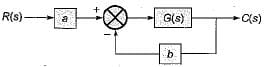Detailed Solution for Test: Feedback Characteristics - Question 1

The closed loop transfer function is given by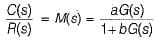∴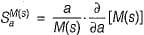Now,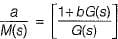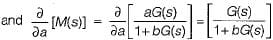∴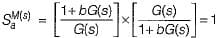Test: Feedback Characteristics - Question 2

### For the system shown in figure below,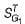is equal to (where, T = closed loop transfer function)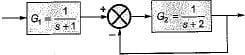Detailed Solution for Test: Feedback Characteristics - Question 2

The CLTF is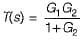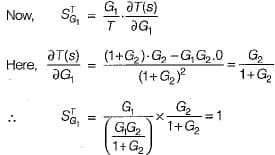Test: Feedback Characteristics - Question 3

### In control system, the feedback

Test: Feedback Characteristics - Question 4

For the closed loop transfer function shown below, E(s)/R(s) is given by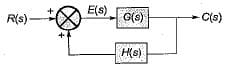Detailed Solution for Test: Feedback Characteristics - Question 4

We have:   E(s) = R(s) + C(s) H(s) ...(i)

Also,   C(s) = E(s) G(s) ...(ii)
From (i) and (ii), we have:
E(s) = R(s) + E(s) G(s) H(s)
or, E(s) (1-G(s)H(s)] = R(s)
or,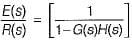Test: Feedback Characteristics - Question 5

Due to positive feedback

Test: Feedback Characteristics - Question 6

Consider the following statements:
1. Closed loop system is less sensitive to variations in feedback path parameters than variations in forward path parameters.
2. Feedback can improve the stability or may be harmful to stability if it is not properly designed and applied.
3. Feedback controls the time response of the system by adjusting the location of poles.

Q. Which of the these statements are correct?

Detailed Solution for Test: Feedback Characteristics - Question 6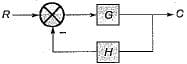For the closed loop control system shown above, let, H = C/R transfer function.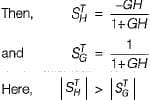Thus, closed loop system is more sensitive to variation in feedback path parameters than variation in forward path parameters. Hence statement-1 is false. Thus, statements 2 and 3 are only correct.

Test: Feedback Characteristics - Question 7

An unity feedback control system has a transfer function of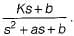The open loop transfer function of this system is

Detailed Solution for Test: Feedback Characteristics - Question 7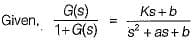∴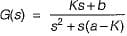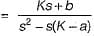Test: Feedback Characteristics - Question 8

Assertion (A): Feedback in control system improves the time response of the system. ​
Reason (R): Time constant of an open loop system is less than the time constant of a closed loop system.

Detailed Solution for Test: Feedback Characteristics - Question 8

Time constant of open loop system is more than that of closed loop system. Lesser is the time constant of the system, faster is its' time response.

Test: Feedback Characteristics - Question 9

For the unity feedback system shown below, if the input to the system is a unit-step signal, then the integral square error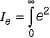(t) dt for infinite value of gain k will be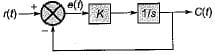Detailed Solution for Test: Feedback Characteristics - Question 9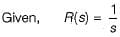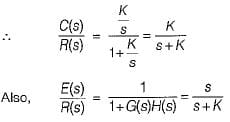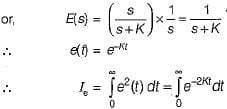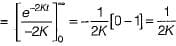Thus, when K = ∞, Ie = 0.

Test: Feedback Characteristics - Question 10

Introduction of feedback to a system

Detailed Solution for Test: Feedback Characteristics - Question 10

Introduction of feed back to a system will increase the stability if negative feedback is introduced while it will reduce the stability if positive feedback is introduced.

## GATE Electrical Engineering (EE) 2024 Mock Test Series

23 docs|285 tests
Information about Test: Feedback Characteristics Page
In this test you can find the Exam questions for Test: Feedback Characteristics solved & explained in the simplest way possible. Besides giving Questions and answers for Test: Feedback Characteristics, EduRev gives you an ample number of Online tests for practice

## GATE Electrical Engineering (EE) 2024 Mock Test Series

23 docs|285 tests